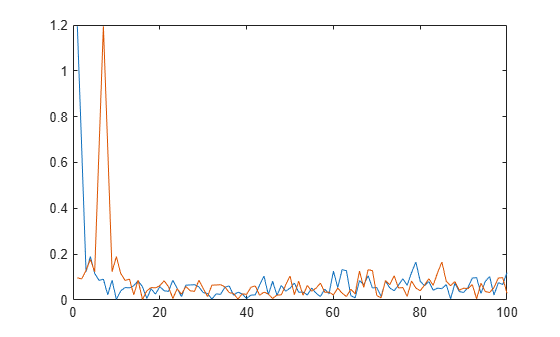# lteULFrameOffset

PUSCH DM-RS uplink subframe timing estimate

## Syntax

``offset = lteULFrameOffset(ue,chs,waveform)``
``````[offset,corr] = lteULFrameOffset(ue,chs,waveform)``````

## Description

example

````offset = lteULFrameOffset(ue,chs,waveform)` performs synchronization using PUSCH DM-RS signals for the time-domain waveform, `waveform`, given UE-specific settings, `ue`, and PUSCH configuration, `chs`.The returned value `offset` indicates the number of samples from the start of the waveform, `waveform`, to the position in that waveform where the first subframe containing the DM-RS begins.`offset` provides subframe timing; frame timing can be achieved by using `offset` with the subframe number, `ue``.``NSubframe`. This information is consistent with real-world operation, since the base station knows when, or in which subframe, to expect uplink transmissions. ```

example

``````[offset,corr] = lteULFrameOffset(ue,chs,waveform)``` also returns a complex matrix `corr`, which is the signal used to extract the timing offset.```

## Examples

collapse all

Synchronization and demodulation of transmission which has been delayed by 5 samples.

Initialize waveform and insert a 5 sample delay.

```ue = lteRMCUL('A3-2'); waveform = lteRMCULTool(ue,[1;0;0;1]); tx = [zeros(5,1); waveform];```

Determine `offset` and demodulate the waveform.

`offset = lteULFrameOffset(ue,ue.PUSCH,tx)`
```offset = 5 ```
`rxGrid = lteSCFDMADemodulate(ue,tx(1+offset:end));`

View the correlation peak for a delayed transmit waveform. The transmission contains PUSCH demodulation reference signal (DM-RS) symbols available for estimating the waveform timing.

UE Configuration

Configure UE-specific settings and generate the transmit waveform.

```ue = lteRMCUL('A3-2'); tx = lteRMCULTool(ue,[1;0;0;1]);```

Determine Offset

Calculate timing offset and return the correlations for the transmit waveform and for a delayed version of the transmit waveform.

```[~,corr] = lteULFrameOffset(ue,ue.PUSCH,tx); txDelayed = [zeros(6,1); tx]; [offset,corrDelayed] = lteULFrameOffset(ue,ue.PUSCH,txDelayed); ```

Plot the correlation data before and after delay is added. Zoom in on the x-axis to view correlation peaks.

```plot(corr) hold on plot(corrDelayed) hold off xlim([0 100])```Correct the timing offset and demodulate the received waveform.

`rxGrid = lteSCFDMADemodulate(ue,txDelayed(1+offset:end));`

## Input Arguments

collapse all

UE-specific settings, specified as a scalar structure with the following fields.

Parameter FieldRequired or OptionalValuesDescription
`NULRB`Required

Scalar integer from 6 to 110

Number of uplink resource blocks. (${N}_{\text{RB}}^{\text{UL}}$)

`NCellID`Required

Integer from 0 to 503

Physical layer cell identity

`NSubframe`Required

0 (default), nonnegative scalar integer

Subframe number

`CyclicPrefixUL`Optional

`'Normal'` (default), `'Extended'`

Cyclic prefix length

`NTxAnts`Optional

1 (default), 2, 4

Number of transmission antennas.

`Hopping`Optional

`'Off'` (default), `'Group'`, or `'Sequence'`

Frequency hopping method.

`SeqGroup`Optional

0 (default), integer from 0 to 29

PUSCH sequence group assignment (ΔSS).

Only used if `NDMRSID` or `NPUSCHID` is absent.

`CyclicShift`Optional

0 (default), integer from 0 to 7

Number of cyclic shifts used for PUSCH DM-RS (yields ${n}_{DMRS}^{\left(1\right)}$).

`NPUSCHID`Optional

0 (default), nonnegative scalar integer from 0 to 509

PUSCH virtual cell identity. If this field is not present, `NCellID` is used for group hopping sequence-shift pattern initialization.

See footnote.

`NDMRSID`Optional

0 (default), nonnegative scalar integer from 0 to 509

DM-RS identity for cyclic shift hopping (${n}_{ID}^{csh_DMRS}$). If this field is not present, `NCellID` is used for cyclic shift hopping initialization.

See footnote.

1. The pseudorandom sequence generator for cyclic shift hopping is initialized according to `NDMRSID`, if present — otherwise it is initialized according to the cell identity `NCellID` and the sequence group assignment `SeqGroup`. Similarly, the sequence-shift pattern for group hopping is initialized according to `NPUSCHID`, if present — otherwise it is initialized according to `NCellID` and `SeqGroup`.

Data Types: `struct`

PUSCH configuration, specified as a scalar structure with the following fields.

Parameter FieldRequired or OptionalValuesDescription
`PRBSet`Required

Integer column vector or two-column matrix

0-based physical resource block indices (PRBs) for the slots of the current PUSCH resource allocation. As a column vector, the resource allocation is the same in both slots of the subframe. As a two-column matrix, it specifies different PRBs for each slot in a subframe.

`NLayers`Optional

1 (default), 2, 3, 4

Number of transmission layers.

`DynCyclicShift`Optional

0 (default), integer from 0 to 7

Cyclic shift for DM-RS (yields ${n}_{DMRS}^{\left(2\right)}$).

`OrthCover`Optional

`'Off'` (default), `'On'`

Applies (`'On'`), or does not apply (`'Off'`), orthogonal cover sequence w (Activate-DMRS-with OCC).

The following field is required only when `ue``.``NTxAnts` is set to 2 or 4.
`PMI`Optional

0 (default), nonnegative scalar integer from 0 to 23.

Scalar precoder matrix indication (PMI) to be used during precoding

Data Types: `struct`

Time-domain waveform, specified as a numeric matrix. `waveform` must be a NS-by-NR matrix, where NS is the number of time-domain samples and NR is the number of receive antennas. `waveform` should be at least one subframe long and contain the DM-RS signals.

Generate `waveform` by SC-FDMA modulation of a resource matrix using the `lteSCFDMAModulate` function, or by using one of the channel model functions, `lteFadingChannel`, `lteHSTChannel`, or `lteMovingChannel`.

Data Types: `double`
Complex Number Support: Yes

## Output Arguments

collapse all

Offset number of samples, returned as a scalar integer. This output is the number of samples from the start of the waveform to the position in that waveform where the first subframe containing the DM-RS begins. `offset` is computed by extracting the timing of the peak of the correlation between `waveform` and internally generated reference waveforms containing DM-RS signals. The correlation is performed separately for each antenna and the antenna with the strongest correlation is used to compute `offset`.

Note

`offset` is the position of `mod(max(abs(corr),LSF))`, where LSF is the subframe length.

Signal used to extract the timing offset, returned as a complex-valued numeric matrix. `corr` has the same dimensions as `waveform`.

## Version History

Introduced in R2014a